Continuous Random Variables

# 28 The Exponential Distribution

The exponential distribution is often concerned with the amount of time until some specific event occurs. For example, the amount of time (beginning now) until an earthquake occurs has an exponential distribution. Other examples include the length of time, in minutes, of long distance business telephone calls, and the amount of time, in months, a car battery lasts. It can be shown, too, that the value of the change that you have in your pocket or purse approximately follows an exponential distribution.

Values for an exponential random variable occur in the following way. There are fewer large values and more small values. For example, marketing studies have shown that the amount of money customers spend in one trip to the supermarket follows an exponential distribution. There are more people who spend small amounts of money and fewer people who spend large amounts of money.

Exponential distributions are commonly used in calculations of product reliability, or the length of time a product lasts.

The random variable for the exponential distribution is continuous and often measures a passage of time, although it can be used in other applications. Typical questions may be, “what is the probability that some event will occur within the next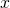hours or days, or what is the probability that some event will occur between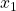hours and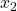hours, or what is the probability that the event will take more thanhours to perform?” In short, the random variable X equals (a) the time between events or (b) the passage of time to complete an action, e.g. wait on a customer. The probability density function is given by: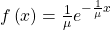where μ is the historical average waiting time.

and has a mean and standard deviation of 1/μ.

An alternative form of the exponential distribution formula recognizes what is often called the decay factor. The decay factor simply measures how rapidly the probability of an event declines as the random variable X increases. When the notation using the decay parameter m is used, the probability density function is presented as: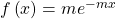where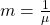In order to calculate probabilities for specific probability density functions, the cumulative density function is used. The cumulative density function (cdf) is simply the integral of the pdf and is: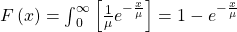Let X = amount of time (in minutes) a postal clerk spends with a customer. The time is known from historical data to have an average amount of time equal to four minutes.

It is given that μ = 4 minutes, that is, the average time the clerk spends with a customer is 4 minutes. Remember that we are still doing probability and thus we have to be told the population parameters such as the mean. To do any calculations, we need to know the mean of the distribution: the historical time to provide a service, for example. Knowing the historical mean allows the calculation of the decay parameter, m.. Therefore,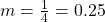.

When the notation used the decay parameter, m, the probability density function is presented as, which is simply the original formula with m substituted for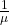, or.

To calculate probabilities for an exponential probability density function, we need to use the cumulative density function. As shown below, the curve for the cumulative density function is:

f(x) = 0.25e–0.25x where x is at least zero and m = 0.25.

For example, f(5) = 0.25e(-0.25)(5) = 0.072. In other words, the function has a value of .072 when x = 5.

The graph is as follows: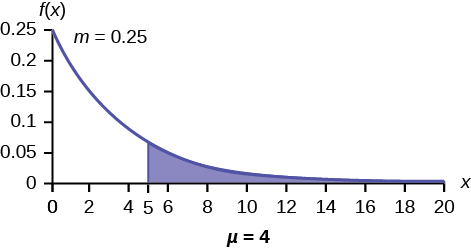Notice the graph is a declining curve. When x = 0,

f(x) = 0.25e(−0.25)(0) = (0.25)(1) = 0.25 = m. The maximum value on the y-axis is always m, one divided by the mean.

Try It

The amount of time spouses shop for anniversary cards can be modeled by an exponential distribution with the average amount of time equal to eight minutes. Write the distribution, state the probability density function, and graph the distribution.

X ~ Exp(0.125); f(x) = 0.125e–0.125x;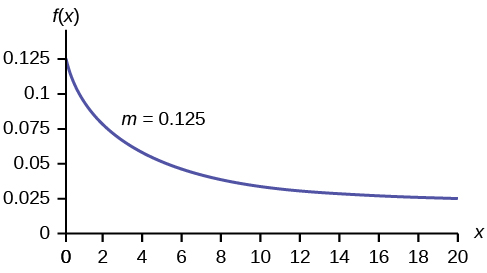a. Using the information in (Figure), find the probability that a clerk spends four to five minutes with a randomly selected customer.

a. Find P(4 < x < 5).
The cumulative distribution function (CDF) gives the area to the left.
P(x < x) = 1 – e–mx
P(x < 5) = 1 – e(–0.25)(5) = 0.7135 and P(x < 4) = 1 – e(–0.25)(4) = 0.6321
P(4 < x < 5)= 0.7135 – 0.6321 = 0.0814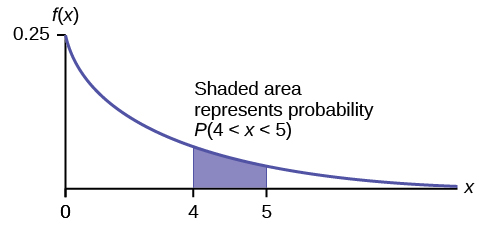Try It

The number of days ahead travelers purchase their airline tickets can be modeled by an exponential distribution with the average amount of time equal to 15 days. Find the probability that a traveler will purchase a ticket fewer than ten days in advance. How many days do half of all travelers wait?

P(x < 10) = 0.4866

50th percentile = 10.40

On the average, a certain computer part lasts ten years. The length of time the computer part lasts is exponentially distributed.

a. What is the probability that a computer part lasts more than 7 years?

a. Let x = the amount of time (in years) a computer part lasts.
μ = 10 so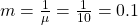Find P(x > 7). Draw the graph.
P(x > 7) = 1 – P(x < 7).
Since P(X < x) = 1 – e–mx then P(X > x) = 1 – ( 1 –e–mx) = e–mx
P(x > 7) = e(–0.1)(7) = 0.4966. The probability that a computer part lasts more than seven years is 0.4966.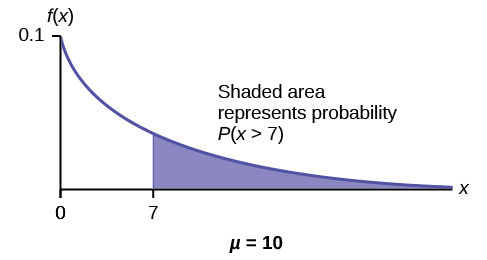b. On the average, how long would five computer parts last if they are used one after another?

b. On the average, one computer part lasts ten years. Therefore, five computer parts, if they are used one right after the other would last, on the average, (5)(10) = 50 years.

d. What is the probability that a computer part lasts between nine and 11 years?

d. Find P(9 < x < 11). Draw the graph.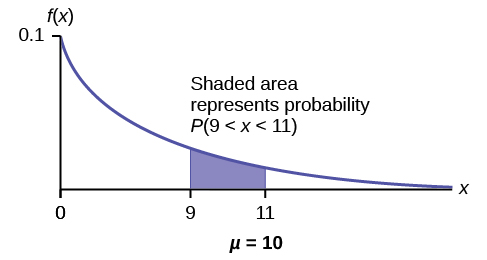P(9 < x < 11) = P(x < 11) – P(x < 9) = (1 – e(–0.1)(11)) – (1 – e(–0.1)(9)) = 0.6671 – 0.5934 = 0.0737. The probability that a computer part lasts between nine and 11 years is 0.0737.

Try It

On average, a pair of running shoes can last 18 months if used every day. The length of time running shoes last is exponentially distributed. What is the probability that a pair of running shoes last more than 15 months? On average, how long would six pairs of running shoes last if they are used one after the other? Eighty percent of running shoes last at most how long if used every day?

P(x > 15) = 0.4346

Six pairs of running shoes would last 108 months on average.

80th percentile = 28.97 months

Suppose that the length of a phone call, in minutes, is an exponential random variable with decay parameter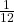. The decay p[parameter is another way to view 1/λ. If another person arrives at a public telephone just before you, find the probability that you will have to wait more than five minutes. Let X = the length of a phone call, in minutes.

What is m, μ, and σ? The probability that you must wait more than five minutes is _______ .

• m =• μ = 12
• σ = 12

P(x > 5) = 0.6592

The time spent waiting between events is often modeled using the exponential distribution. For example, suppose that an average of 30 customers per hour arrive at a store and the time between arrivals is exponentially distributed.

1. On average, how many minutes elapse between two successive arrivals?
2. When the store first opens, how long on average does it take for three customers to arrive?
3. After a customer arrives, find the probability that it takes less than one minute for the next customer to arrive.
4. After a customer arrives, find the probability that it takes more than five minutes for the next customer to arrive.
5. Is an exponential distribution reasonable for this situation?
1. Since we expect 30 customers to arrive per hour (60 minutes), we expect on average one customer to arrive every two minutes on average.
2. Since one customer arrives every two minutes on average, it will take six minutes on average for three customers to arrive.
3. Let X = the time between arrivals, in minutes. By part a, μ = 2, so m == 0.5.
The cumulative distribution function is P(X < x) = 1 – e(-0.5)(x)
Therefore P(X < 1) = 1 – e(–0.5)(1) = 0.3935.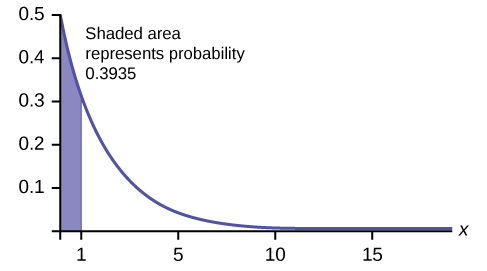4. P(X > 5) = 1 – P(X < 5) = 1 – (1 – e(-0.5)(5)) = e–2.5 ≈ 0.0821.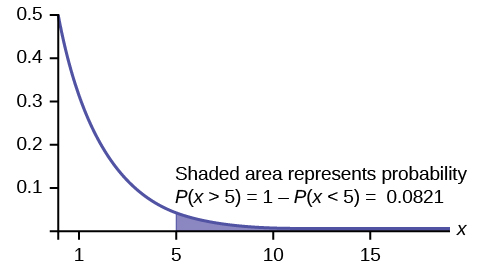5. This model assumes that a single customer arrives at a time, which may not be reasonable since people might shop in groups, leading to several customers arriving at the same time. It also assumes that the flow of customers does not change throughout the day, which is not valid if some times of the day are busier than others.

### Memorylessness of the Exponential Distribution

Recall that the amount of time between customers for the postal clerk discussed earlier is exponentially distributed with a mean of two minutes. Suppose that five minutes have elapsed since the last customer arrived. Since an unusually long amount of time has now elapsed, it would seem to be more likely for a customer to arrive within the next minute. With the exponential distribution, this is not the case–the additional time spent waiting for the next customer does not depend on how much time has already elapsed since the last customer. This is referred to as the memoryless property. The exponential and geometric probability density functions are the only probability functions that have the memoryless property. Specifically, the memoryless property says that

P (X > r + t | X > r) = P (X > t) for all r ≥ 0 and t ≥ 0

For example, if five minutes have elapsed since the last customer arrived, then the probability that more than one minute will elapse before the next customer arrives is computed by using r = 5 and t = 1 in the foregoing equation.

P(X > 5 + 1 | X > 5) = P(X > 1) =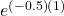= 0.6065.

This is the same probability as that of waiting more than one minute for a customer to arrive after the previous arrival.

The exponential distribution is often used to model the longevity of an electrical or mechanical device. In (Figure), the lifetime of a certain computer part has the exponential distribution with a mean of ten years. The memoryless property says that knowledge of what has occurred in the past has no effect on future probabilities. In this case it means that an old part is not any more likely to break down at any particular time than a brand new part. In other words, the part stays as good as new until it suddenly breaks. For example, if the part has already lasted ten years, then the probability that it lasts another seven years is P(X > 17|X > 10) = P(X > 7) = 0.4966, where the vertical line is read as “given”.

Refer back to the postal clerk again where the time a postal clerk spends with his or her customer has an exponential distribution with a mean of four minutes. Suppose a customer has spent four minutes with a postal clerk. What is the probability that he or she will spend at least an additional three minutes with the postal clerk?

The decay parameter of X is m == 0.25, so XExp(0.25).

The cumulative distribution function is P(X < x) = 1 – e–0.25x.

We want to find P(X > 7|X > 4). The memoryless property says that P(X > 7|X > 4) = P (X > 3), so we just need to find the probability that a customer spends more than three minutes with a postal clerk.

This is P(X > 3) = 1 – P (X < 3) = 1 – (1 – e–0.25⋅3) = e–0.75 ≈ 0.4724.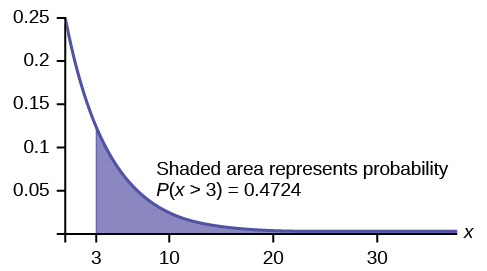### Relationship between the Poisson and the Exponential Distribution

There is an interesting relationship between the exponential distribution and the Poisson distribution. Suppose that the time that elapses between two successive events follows the exponential distribution with a mean of μ units of time. Also assume that these times are independent, meaning that the time between events is not affected by the times between previous events. If these assumptions hold, then the number of events per unit time follows a Poisson distribution with mean μ. Recall that if X has the Poisson distribution with mean μ, then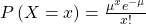.

The formula for the exponential distribution: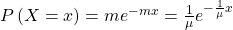Where m = the rate parameter, or μ = average time between occurrences.

We see that the exponential is the cousin of the Poisson distribution and they are linked through this formula. There are important differences that make each distribution relevant for different types of probability problems.

First, the Poisson has a discrete random variable, x, where time; a continuous variable is artificially broken into discrete pieces. We saw that the number of occurrences of an event in a given time interval, x, follows the Poisson distribution.

For example, the number of times the telephone rings per hour. By contrast, the time between occurrences follows the exponential distribution. For example. The telephone just rang, how long will it be until it rings again? We are measuring length of time of the interval, a continuous random variable, exponential, not events during an interval, Poisson.

#### The Exponential Distribution v. the Poisson Distribution

A visual way to show both the similarities and differences between these two distributions is with a time line.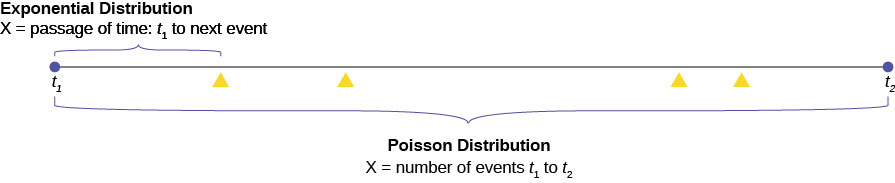The random variable for the Poisson distribution is discrete and thus counts events during a given time period, t1 to t2 on (Figure), and calculates the probability of that number occurring. The number of events, four in the graph, is measured in counting numbers; therefore, the random variable of the Poisson is a discrete random variable.

The exponential probability distribution calculates probabilities of the passage of time, a continuous random variable. In (Figure) this is shown as the bracket from t1 to the next occurrence of the event marked with a triangle.

Classic Poisson distribution questions are “how many people will arrive at my checkout window in the next hour?”.

Classic exponential distribution questions are “how long it will be until the next person arrives,” or a variant, “how long will the person remain here once they have arrived?”.

Again, the formula for the exponential distribution is: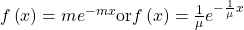We see immediately the similarity between the exponential formula and the Poisson formula.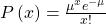Both probability density functions are based upon the relationship between time and exponential growth or decay. The “e” in the formula is a constant with the approximate value of 2.71828 and is the base of the natural logarithmic exponential growth formula. When people say that something has grown exponentially this is what they are talking about.

An example of the exponential and the Poisson will make clear the differences been the two. It will also show the interesting applications they have.

Poisson DistributionSuppose that historically 10 customers arrive at the checkout lines each hour. Remember that this is still probability so we have to be told these historical values. We see this is a Poisson probability problem.

We can put this information into the Poisson probability density function and get a general formula that will calculate the probability of any specific number of customers arriving in the next hour.

The formula is for any value of the random variable we chose, and so the x is put into the formula. This is the formula: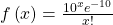As an example, the probability of 15 people arriving at the checkout counter in the next hour would be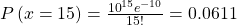Here we have inserted x = 15 and calculated the probability that in the next hour 15 people will arrive is .061.

Exponential DistributionIf we keep the same historical facts that 10 customers arrive each hour, but we now are interested in the service time a person spends at the counter, then we would use the exponential distribution. The exponential probability function for any value of x, the random variable, for this particular checkout counter historical data is: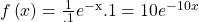To calculate µ, the historical average service time, we simply divide the number of people that arrive per hour, 10 , into the time period, one hour, and have µ = 0.1. Historically, people spend 0.1 of an hour at the checkout counter, or 6 minutes. This explains the .1 in the formula.

There is a natural confusion with µ in both the Poisson and exponential formulas. They have different meanings, although they have the same symbol. The mean of the exponential is one divided by the mean of the Poisson. If you are given the historical number of arrivals you have the mean of the Poisson. If you are given an historical length of time between events you have the mean of an exponential.

Continuing with our example at the checkout clerk; if we wanted to know the probability that a person would spend 9 minutes or less checking out, then we use this formula. First, we convert to the same time units which are parts of one hour. Nine minutes is 0.15 of one hour. Next we note that we are asking for a range of values. This is always the case for a continuous random variable. We write the probability question as: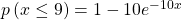We can now put the numbers into the formula and we have our result.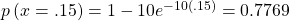The probability that a customer will spend 9 minutes or less checking out is 0.7769.

We see that we have a high probability of getting out in less than nine minutes and a tiny probability of having 15 customers arriving in the next hour.

### Chapter Review

If X has an exponential distribution with mean μ, then the decay parameter is m =. The probability density function of X is f(x) = me-mx (or equivalently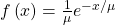. The cumulative distribution function of X is P(Xx) = 1 – emx.

### Formula Review

• pdf: f(x) = me(–mx) where x ≥ 0 and m > 0
• cdf: P(Xx) = 1 – e(–mx)
• mean µ =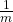• standard deviation σ = µ
• P(X > x) = e(–mx)
• P(a < X < b) = e(–ma)e(–mb)
• Poisson probability:with mean and variance of μ

### References

Data from the United States Census Bureau.

Data from World Earthquakes, 2013. Available online at http://www.world-earthquakes.com/ (accessed June 11, 2013).

“No-hitter.” Baseball-Reference.com, 2013. Available online at http://www.baseball-reference.com/bullpen/No-hitter (accessed June 11, 2013).

Zhou, Rick. “Exponential Distribution lecture slides.” Available online at www.public.iastate.edu/~riczw/stat330s11/lecture/lec13.pdf‎ (accessed June 11, 2013).

Use the following information to answer the next ten exercises. A customer service representative must spend different amounts of time with each customer to resolve various concerns. The amount of time spent with each customer can be modeled by the following distribution: X ~ Exp(0.2)

What type of distribution is this?

Are outcomes equally likely in this distribution? Why or why not?

No, outcomes are not equally likely. In this distribution, more people require a little bit of time, and fewer people require a lot of time, so it is more likely that someone will require less time.

What is m? What does it represent?

What is the mean?

five

What is the standard deviation?

State the probability density function.

f(x) = 0.2e-0.2x

Graph the distribution.

Find P(2 < x < 10).

0.5350

Find P(x > 6).

Find the 70th percentile.

6.02

Use the following information to answer the next seven exercises. A distribution is given as X ~ Exp(0.75).

What is m?

What is the probability density function?

f(x) = 0.75e-0.75x

What is the cumulative distribution function?

Draw the distribution.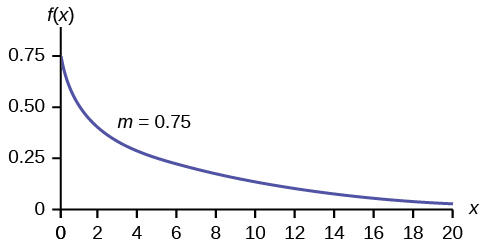Find P(x < 4).

Find the 30th percentile.

0.4756

Find the median.

Which is larger, the mean or the median?

The mean is larger. The mean is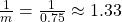, which is greater than 0.9242.

Use the following information to answer the next 16 exercises. Carbon-14 is a radioactive element with a half-life of about 5,730 years. Carbon-14 is said to decay exponentially. The decay rate is 0.000121. We start with one gram of carbon-14. We are interested in the time (years) it takes to decay carbon-14.

What is being measured here?

Are the data discrete or continuous?

continuous

In words, define the random variable X.

What is the decay rate (m)?

m = 0.000121

The distribution for X is ______.

Find the amount (percent of one gram) of carbon-14 lasting less than 5,730 years. This means, find P(x < 5,730).

1. Sketch the graph, and shade the area of interest.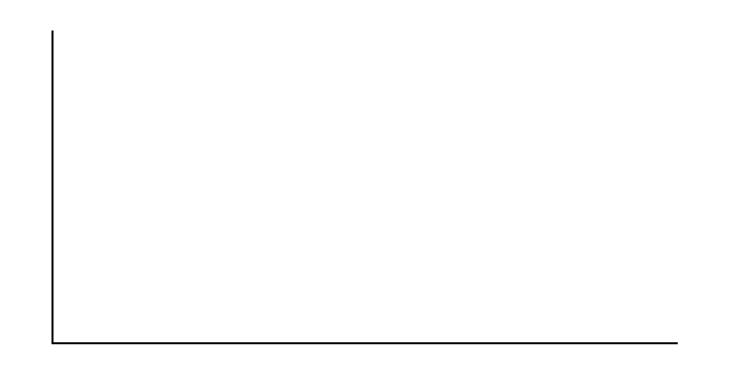2. Find the probability. P(x < 5,730) = __________
1. Check student’s solution
2. P(x < 5,730) = 0.5001

Find the percentage of carbon-14 lasting longer than 10,000 years.

1. Sketch the graph, and shade the area of interest.2. Find the probability. P(x > 10,000) = ________

Thirty percent (30%) of carbon-14 will decay within how many years?

1. Sketch the graph, and shade the area of interest.2. Find the value k such that P(x < k) = 0.30.
1. Check student’s solution.
2. k = 2947.73

### Homework

Suppose that the length of long distance phone calls, measured in minutes, is known to have an exponential distribution with the average length of a call equal to eight minutes.

1. Define the random variable. X = ________________.
2. Is X continuous or discrete?
3. μ = ________
4. σ = ________
5. Draw a graph of the probability distribution. Label the axes.
6. Find the probability that a phone call lasts less than nine minutes.
7. Find the probability that a phone call lasts more than nine minutes.
8. Find the probability that a phone call lasts between seven and nine minutes.
9. If 25 phone calls are made one after another, on average, what would you expect the total to be? Why?

Suppose that the useful life of a particular car battery, measured in months, decays with parameter 0.025. We are interested in the life of the battery.

1. Define the random variable. X = _________________________________.
2. Is X continuous or discrete?
3. On average, how long would you expect one car battery to last?
4. On average, how long would you expect nine car batteries to last, if they are used one after another?
5. Find the probability that a car battery lasts more than 36 months.
6. Seventy percent of the batteries last at least how long?
1. X = the useful life of a particular car battery, measured in months.
2. X is continuous.
3. 40 months
4. 360 months
5. 0.4066
6. 14.27

The percent of persons (ages five and older) in each state who speak a language at home other than English is approximately exponentially distributed with a mean of 9.848. Suppose we randomly pick a state.

1. Define the random variable. X = _________________________________.
2. Is X continuous or discrete?
3. μ = ________
4. σ = ________
5. Draw a graph of the probability distribution. Label the axes.
6. Find the probability that the percent is less than 12.
7. Find the probability that the percent is between eight and 14.
8. The percent of all individuals living in the United States who speak a language at home other than English is 13.8.
1. Why is this number different from 9.848%?
2. What would make this number higher than 9.848%?

The time (in years) after reaching age 60 that it takes an individual to retire is approximately exponentially distributed with a mean of about five years. Suppose we randomly pick one retired individual. We are interested in the time after age 60 to retirement.

1. Define the random variable. X = _________________________________.
2. Is X continuous or discrete?
3. μ = ________
4. σ = ________
5. Draw a graph of the probability distribution. Label the axes.
6. Find the probability that the person retired after age 70.
7. Do more people retire before age 65 or after age 65?
8. In a room of 1,000 people over age 80, how many do you expect will NOT have retired yet?
1. X = the time (in years) after reaching age 60 that it takes an individual to retire
2. X is continuous.
3. five
4. five
5. Check student’s solution.
6. 0.1353
7. before
8. 18.3

The cost of all maintenance for a car during its first year is approximately exponentially distributed with a mean of ?150.

1. Define the random variable. X = _________________________________.
2. μ = ________
3. σ = ________
4. Draw a graph of the probability distribution. Label the axes.
5. Find the probability that a car required over ?300 for maintenance during its first year.

Use the following information to answer the next three exercises. The average lifetime of a certain new cell phone is three years. The manufacturer will replace any cell phone failing within two years of the date of purchase. The lifetime of these cell phones is known to follow an exponential distribution.

The decay rate is:

1. 0.3333
2. 0.5000
3. 2
4. 3

a

What is the probability that a phone will fail within two years of the date of purchase?

1. 0.8647
2. 0.4866
3. 0.2212
4. 0.9997

What is the median lifetime of these phones (in years)?

1. 0.1941
2. 1.3863
3. 2.0794
4. 5.5452

c

At a 911 call center, calls come in at an average rate of one call every two minutes. Assume that the time that elapses from one call to the next has the exponential distribution.

1. On average, how much time occurs between five consecutive calls?
2. Find the probability that after a call is received, it takes more than three minutes for the next call to occur.
3. Ninety-percent of all calls occur within how many minutes of the previous call?
4. Suppose that two minutes have elapsed since the last call. Find the probability that the next call will occur within the next minute.
5. Find the probability that less than 20 calls occur within an hour.

In major league baseball, a no-hitter is a game in which a pitcher, or pitchers, doesn’t give up any hits throughout the game. No-hitters occur at a rate of about three per season. Assume that the duration of time between no-hitters is exponential.

1. What is the probability that an entire season elapses with a single no-hitter?
2. If an entire season elapses without any no-hitters, what is the probability that there are no no-hitters in the following season?
3. What is the probability that there are more than 3 no-hitters in a single season?

Let X = the number of no-hitters throughout a season. Since the duration of time between no-hitters is exponential, the number of no-hitters per season is Poisson with mean λ = 3.
Therefore, (X = 0) =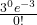= e–3 ≈ 0.0498

NOTE

You could let T = duration of time between no-hitters. Since the time is exponential and there are 3 no-hitters per season, then the time between no-hitters isseason. For the exponential, µ =.
Therefore, m == 3 and TExp(3).

1. The desired probability is P(T > 1) = 1 – P(T < 1) = 1 – (1 – e–3) = e–3 ≈ 0.0498.
2. Let T = duration of time between no-hitters. We find P(T > 2|T > 1), and by the memoryless property this is simply P(T > 1), which we found to be 0.0498 in part a.
3. Let X = the number of no-hitters is a season. Assume that X is Poisson with mean λ = 3. Then P(X > 3) = 1 – P(X ≤ 3) = 0.3528.

During the years 1998–2012, a total of 29 earthquakes of magnitude greater than 6.5 have occurred in Papua New Guinea. Assume that the time spent waiting between earthquakes is exponential.

1. What is the probability that the next earthquake occurs within the next three months?
2. Given that six months has passed without an earthquake in Papua New Guinea, what is the probability that the next three months will be free of earthquakes?
3. What is the probability of zero earthquakes occurring in 2014?
4. What is the probability that at least two earthquakes will occur in 2014?

According to the American Red Cross, about one out of nine people in the U.S. have Type B blood. Suppose the blood types of people arriving at a blood drive are independent. In this case, the number of Type B blood types that arrive roughly follows the Poisson distribution.

1. If 100 people arrive, how many on average would be expected to have Type B blood?
2. What is the probability that over 10 people out of these 100 have type B blood?
3. What is the probability that more than 20 people arrive before a person with type B blood is found?
1.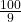= 11.11
2. P(X > 10) = 1 – P(X ≤ 10) = 1 – Poissoncdf(11.11, 10) ≈ 0.5532.
3. The number of people with Type B blood encountered roughly follows the Poisson distribution, so the number of people X who arrive between successive Type B arrivals is roughly exponential with mean μ = 9 and m =. The cumulative distribution function of X is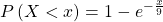. Thus hus, P(X > 20) = 1 – P(X ≤ 20) =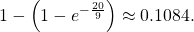Note

We could also deduce that each person arriving has a 8/9 chance of not having Type B blood. So the probability that none of the first 20 people arrive have Type B blood is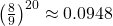. (The geometric distribution is more appropriate than the exponential because the number of people between Type B people is discrete instead of continuous.)

A web site experiences traffic during normal working hours at a rate of 12 visits per hour. Assume that the duration between visits has the exponential distribution.

1. Find the probability that the duration between two successive visits to the web site is more than ten minutes.
2. The top 25% of durations between visits are at least how long?
3. Suppose that 20 minutes have passed since the last visit to the web site. What is the probability that the next visit will occur within the next 5 minutes?
4. Find the probability that less than 7 visits occur within a one-hour period.

At an urgent care facility, patients arrive at an average rate of one patient every seven minutes. Assume that the duration between arrivals is exponentially distributed.

1. Find the probability that the time between two successive visits to the urgent care facility is less than 2 minutes.
2. Find the probability that the time between two successive visits to the urgent care facility is more than 15 minutes.
3. If 10 minutes have passed since the last arrival, what is the probability that the next person will arrive within the next five minutes?
4. Find the probability that more than eight patients arrive during a half-hour period.

Let T = duration (in minutes) between successive visits. Since patients arrive at a rate of one patient every seven minutes, μ = 7 and the decay constant is m =. The cdf is P(T < t) =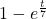1. P(T < 2) = 1 –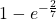≈ 0.2485.
2. P(T > 15) =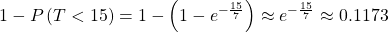.
3. P(T > 15|T > 10) = P(T > 5) =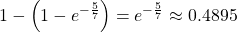.
4. Let X = # of patients arriving during a half-hour period. Then X has the Poisson distribution with a mean of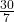, X ∼ Poisson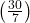. Find P(X > 8) = 1 – P(X ≤ 8) ≈ 0.0311.

### Key Terms

decay parameter
The decay parameter describes the rate at which probabilities decay to zero for increasing values of x. It is the value m in the probability density function f(x) = me(-mx) of an exponential random variable. It is also equal to m =, where μ is the mean of the random variable.
memoryless property
For an exponential random variable X, the memoryless property is the statement that knowledge of what has occurred in the past has no effect on future probabilities. This means that the probability that X exceeds x + t, given that it has exceeded x, is the same as the probability that X would exceed t if we had no knowledge about it. In symbols we say that P(X > x + t|X > x) = P(X > t).
Poisson distribution
If there is a known average of μ events occurring per unit time, and these events are independent of each other, then the number of events X occurring in one unit of time has the Poisson distribution. The probability of x events occurring in one unit time is equal to.